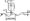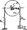# Mechanical Vibrations December 2011

Answer any FIVE full questions, selecting at least TWO questions from each part

PART-A

1 a.Split the harmonic motion x = 10 sin (wt + j^), into two harmonic motions one having a phase angle of zero and the other of 45°.Use both analytical and graphical methods.

b. An unknown weight W, added to an unknown spring K has a natural frequency 95 cycles/min. When 5N is added to W, the natural frequency is lowered to 75 cycles/min. Determine the unknown weight and the spring constant.

c. The ratio K/m of a spring – mass system is given as 4.0. If the mass is deflected 2cm down measured from the equilibrium position and given an upward velocity of 8cm/s, determine its amplitude and maximum acceleration.

2  a. A uniform rigid bar is pivoted at one end and connected symmetrically by two springs at the other end as shown in fig. Q 2(a). Assume that the mass of the bar is m and that the springs are un stretched when the bar is vertical. Determine the natural frequency of oscillation of the bar for small displacements.  Is  there any limitations on the value of K? Discuss.

b. Use the energy method to find the natural frequency of oscillation of the homogeneous cylinder shown in fig.Q2(b).Fig. Q2 (b)

3 a. Derive an expression for the oscillatory motion of a spring-mass-damper system.

b.  The ratio of successive amplitudes of a viscously damped single degree of freedom system is found to be 18:1. Determine the ratio of successive amplitudes if the amount of damping is halved.

c.   Define the toms : i) Damping factor ii) Logarithmic decrement.

4 a. Figure Q4(a) shows a simple model of a motor vehicle that can vibrate in the vertical direction, while traveling over a rough road. The vehicle has a mass of 1200 kg. The suspension system has a spring constant of 400kN/m and a damping factor of § = 0.5. If the vehicle speed is 20km/hr, determine the displacement amplitude of the vehicle. The road surface varies sinusoidally with an amplitude of Y = 0.05m and a wave length of 6m.Fig. Q4 (a)

b. A centrifugal fan weighs 450N and has a rotating imbalance of 2.25 N-m. when dampers having damping factor § = 0,2 are used, specify the springs for mounting, such that, only 10% of the unbalanced force is transmitted to the floor. Also, determine the magnitude of the transmitted force. The fan is running at constant speed of 1000 rpm.

PART-B

5 a.Write a brief note on : i) Seismic instrument ii) Critical speed of shaft.

b.  A vibrometer, whose damping is negligible, is employed to find the magnitude of vibration of a machine structure. It gives a reading of the relative displacement of 0.05mm. The natural frequency of the vibrometer is given as 300 cpm and the machine is running at 100 rpm. What will be the magnitude of displacement, velocity and acceleration of the vibrating machine part?

c.   A shaft, carrying a rotor of weight 450N and eccentricity 2.54mm rotates at 1200 rpm. Determine i) the steady state whirl amplitude and ii) the maximum whirl amplitude during start up conditions of the system. Assume the stiffness of the shaft as 36000N/m and the external damping ratio as 0.1.

6 a. Find the natural frequencies of the system shown in fig.Q6(a). Assume that there is no slip between the cord and the cylinder Ki = 40 N/m , K.2 = 60N/m , mi = 2kg , ni2= 10kg. Fig.Q6(a)

b,  A section of pipe pertaining to a certain machine, vibrates with a large amplitude at a compressor speed of 220 rpm. For analyzing this system, a spring – mass system was suspended from the pipe to act as an absorber. A 1 kg absorber mass tuned to 220 cpm resulted in two resonant frequencies of 188 and 258 cpm. What must be the mass and the spring stiffness of the absorber if the resonant frequencies are to be outside the range of 150 to 310 cpm?

7 a. Derive the differential equation of motion for the lateral vibrations of beams. Obtain the general solution to the differential equations.

b.  State the boundary conditions for determining the normal function in beams with different end fixity conditions.

8. a. A shaft of negligible weight 6cm in diameter and 5m long is simply supported at the ends and carries four weights of 50kg each, at equal distances over the length of the shaft. Find the frequency of vibration by Dun kerley’s method. Take E = 2 * 10″ N/m2. (10 Marks)

b.  Use Holzer’s method to determine the natural frequencies of the spring – mass system shown in fig. Q8(b) .